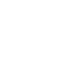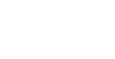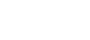# Determination of Darcy’s and Chezy’s Constant for Pipe FlowReading time: 1 minute

OBJECTIVE: i. To determine the Darcy’s and Chezy’s constant for the given pipes, ii. To plot the following graph:
• Darcy’s constant ‘f’ vs Reynold’s number.
• Chezy’s constant ‘C’ vs Reynold’s number
EQUIPMENT: a) Pipes of 15, 20, 25 and 32 mm diameters ‘D’ of length ‘L’ 3 m with flow control valve in each pipe. Upstream and down stream pressure feed pipes as manifold are provided for the measurement of pressure head with control valves situated on a common place for easy operation. b) measuring tank size 0.6 x 0.6 x 0.8 metre with overflow arrangement, gauge glass, scale arrangement and drain valve. c) U tube Differential Mercury Manometer of Range 0.5 m and Scale graduations: 1mm to measure the loss of head. c) Stop watch. BASICS: When water flows through a pipe, a velocity gradient and hence shear stress is produced in the water due to viscous action causing loss of energy. This loss is known as frictional loss. Allow water to flow through the given pipe and measure the head loss due to friction as HHg cm of mercury using the manometer connected at a known distance of L cm. The actual discharge Qa through the pipe is determined by noting the time for collecting h cm of water in the measuring tank. Actual dischargeWhere a - Area of cross-section of measuring tank in cm2. H - Height difference of water in measuring tank in cm. Tm - The mean time to collect wate for a height difference of h cm, measured in seconds (tm = 60 to 120 s) Darcys’ constant, f can be calculated from the relationship. Head lossWhere Hw - HHg * 12.6 cm of water. L - Test length in cm. V - Velocity of water inside the pipeD - Inside diameter of pipe Chezy’s constant C can be calculated from the relationshipWhere V - Velocity of water inside the pipe line cm/s. i - Hydraulic gradient, Hw / L m - D/4 - Hydraulic mean depth (ie. Cross sectional area / wetted perimeter). Reynold’s number for flow through the pipe line is calculated as, Re = (? / ? ) V D Where, ? - Mass density of water ( 1000 kg/m3 at 300 C.) V - Velocity of water inside the pipe D - Diameter of pipe ? -Absolute (dynamic) viscosity of water and is equal to7.82 * 10 - 4 kg/m.s. at 300C. PROCEDURE: i. Connect the mercury manometer to the pressure tappings at the ends of the test length of pipe and close all the valves (pipe line & manometer). ii. Open the needle valves of the manometer & pressure tapping. Ensure that the manometer is primed. iii. Open the inlet valve fully and adjust the outlet valve of the selected pipeline in which the experiment has to be conducted, to note the maximum possible head difference (HHg) of the mercury column. Divide the value (HHg) by seven to fix the steps in the pressure difference for the seven sets of readings. iv. Adjust the outlet valve to get the required pressure difference in the manometer and note down the readings of manometer. v. Note the time in seconds to collect water for a rise of h cm in the measuring tank twice as t1 and t2. If the difference in readings exceeds 10%, take a third reading within the range. vi. Repeat the experiment for different manometer readings. Calculation and graph: Prepare detailed sample calculation for one set of readings as explained in the principle. Calculate the remaining sets of readings and tabulate them. Draw the required graphs using the data tabulated with suitable scale i) Darcy’s constant ‘f’ vs Reynold’s number ii) Chezy’s constant ‘C’ vs Reynold’s number MAINTENANCE: After completing the experiment close the inlet valve and open all the gate valves & needle valves then make them closed. Drain the water from measuring tank after completing the experiment Drain the mercury from manometer by opening the drain plug provided at bottom after two/three months and clean the glass tubes with soap water then fix the drain plug and pour the mercury.
 Sl.No Manometer reading, in mm of Hg Head loss,   mtr of water,Hw Time for 10 cm raise of water level Actual Discharge Qa  m3/s Velocity V m/s Darcy’s Coefficient of friction f Hydraulic gradient, i Chezy’s Constant, C Reynold’s number R h1 h2 h1-h2 t1 t2 tm 1     2     3     4     5     6     7
OBSERVATION: RESULTS: INFERENCE: